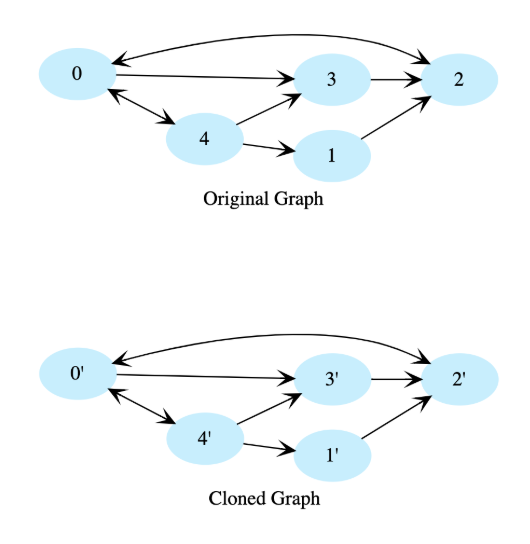# Clone a Directed Graph

## Problem Statement

Given the root node of a directed graph, clone this graph by creating its deep copy so that the cloned graph has the same vertices and edges as the original graph.

Let’s look at the below graphs as an example. If the input graph is G = (V, E) where V is set of vertices and E is set of edges, then the output graph (cloned graph) G’ = (V’, E’) such that V = V’ and E = E’.

Note: We are assuming that all vertices are reachable from the root vertex. i.e. we have a connected graph.## Hint

• Hash table
• Depth first traversal

## Try it yourself

Node* clone(Node* root) {  //TODO: Write - Your - Code   return root;}

## Solution

struct Node {  int data;  list<Node*> neighbors;  Node(int d) : data(d) {}};Node* clone_rec(Node* root,         unordered_map<Node*,         Node*>& nodes_completed) {    if (root == nullptr) {    return nullptr;  }  Node* pNew = new Node(root->data);  nodes_completed[root] = pNew;    for (Node* p : root->neighbors) {        auto x = nodes_completed.find(p);        if (x == nodes_completed.end()){      pNew->neighbors.push_back(clone_rec(p, nodes_completed));    } else {      pNew->neighbors.push_back(x->second /*value*/);    }  }    return pNew;}Node* clone(Node* root) {  unordered_map<Node*, Node*> nodes_completed;  return clone_rec(root, nodes_completed);}// this is un-directed graph i.e.// if there is an edge from x to y// that means there must be an edge from y to x// and there is no edge from a node to itself// hence there can maximim of (nodes * nodes - nodes) / 2 edgesin this graphvoid create_test_graph_undirected(int nodes, int edges, vector<Node*>& vertices) {  for (int i = 0; i < nodes; ++i) {    vertices.push_back(new Node(i));  }  vector<pair<int, int>> all_edges;  for (int i = 0; i < vertices.size(); ++i) {    for (int j = i + 1; j < vertices.size(); ++j) {      all_edges.push_back(pair<int, int>(i, j));    }  }  std::random_shuffle(all_edges.begin(), all_edges.end());  for (int i = 0; i < edges && i < all_edges.size(); ++i) {    pair<int, int>& edge = all_edges[i];    vertices[edge.first]->neighbors.push_back(vertices[edge.second]);    vertices[edge.second]->neighbors.push_back(vertices[edge.first]);  }}void print_graph(vector<Node*>& vertices) {  for (Node* n : vertices) {    cout << n->data << ": {";    for (Node* t : n->neighbors) {      cout << t->data << " ";    }    cout << "}" << endl;  }}void print_graph(Node* root, unordered_set<Node*>& visited_nodes) {  if (root == nullptr || visited_nodes.find(root) != visited_nodes.end()) {    return;  }  visited_nodes.insert(root);  cout << root->data << ": {";  for (Node* n : root->neighbors) {    cout << n->data << " ";  }  cout << "}" << endl;  for (Node* n : root->neighbors) {    print_graph(n, visited_nodes);  }}void print_graph(Node* root) {  unordered_set<Node*> visited_nodes;  print_graph(root, visited_nodes);}int main() {  vector<Node*> vertices;  create_test_graph_undirected(7, 18, vertices);    print_graph(vertices);  Node* cp = clone(vertices);  cout << endl << "After copy" << endl;  print_graph(cp);    return 0;}

## Solution Explanation

Linear, O(n).

### Memory Complexity

Logarithmic, O(n). ‘n’ is the number of vertices in the graph.

### Solution Breakdown

We use depth-first traversal and create a copy of each node while traversing the graph. To avoid getting stuck in cycles, we’ll use a hashtable to store each completed node and will not revisit nodes that exist in the hashtable. The hashtable key will be a node in the original graph, and its value will be the corresponding node in cloned graph.

Practice problems like this and many more by checking out our Grokking the Coding Interview: Patterns for Coding Questions course!

Learn in-demand tech skills in half the time